# Chapter 8 Chemical Reactions 1 Describing Chemical Reactions

• Slides: 53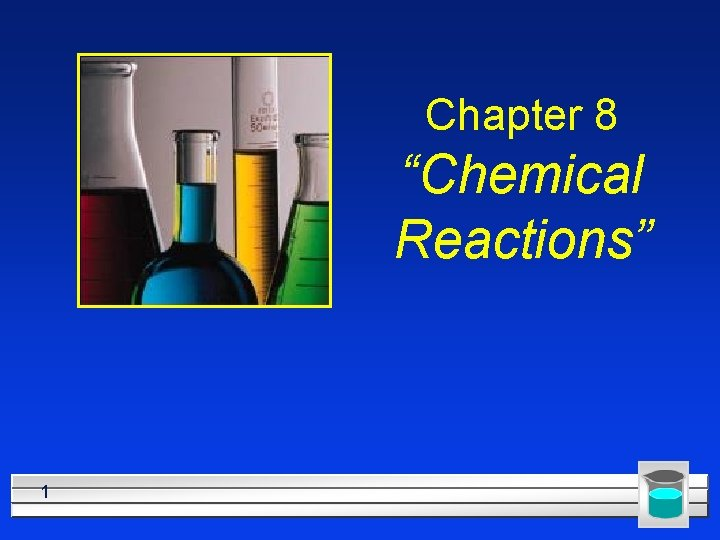Chapter 8 “Chemical Reactions” 1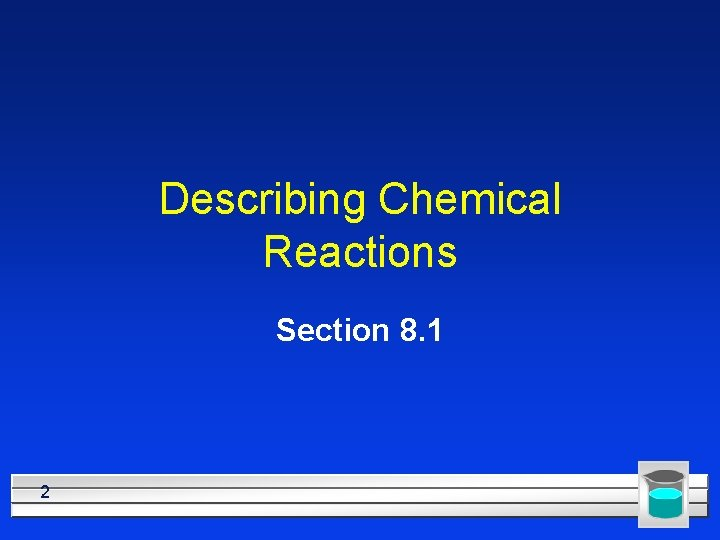Describing Chemical Reactions Section 8. 1 2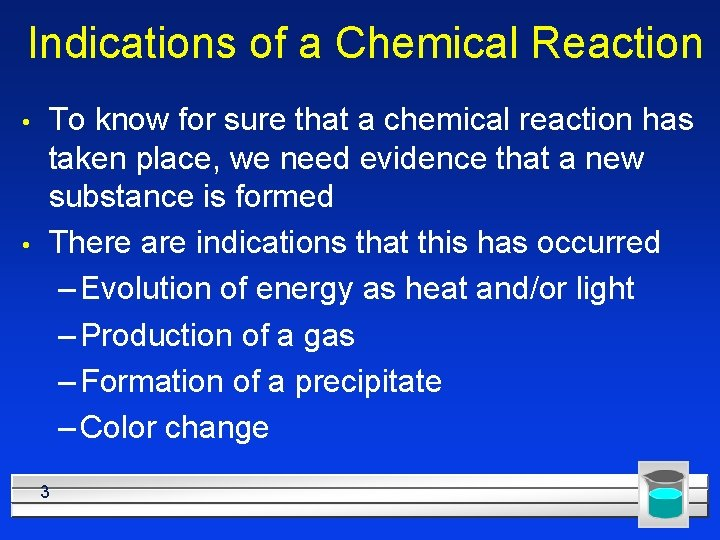Indications of a Chemical Reaction • • To know for sure that a chemical reaction has taken place, we need evidence that a new substance is formed There are indications that this has occurred – Evolution of energy as heat and/or light – Production of a gas – Formation of a precipitate – Color change 3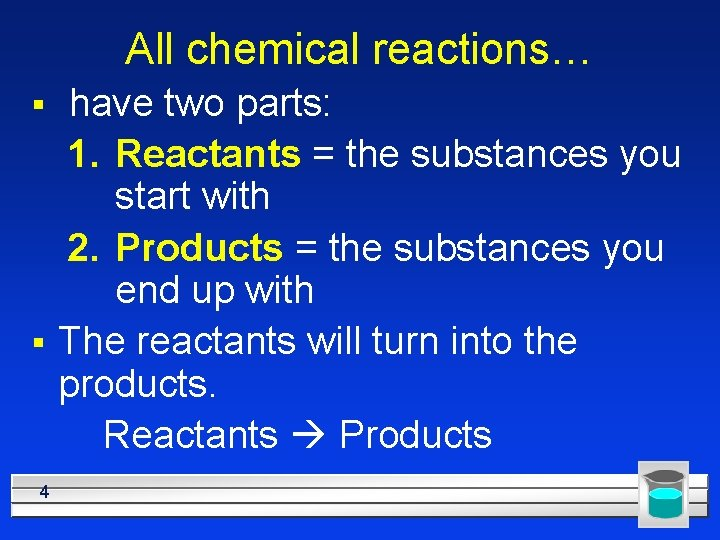All chemical reactions… § § 4 have two parts: 1. Reactants = the substances you start with 2. Products = the substances you end up with The reactants will turn into the products. Reactants Products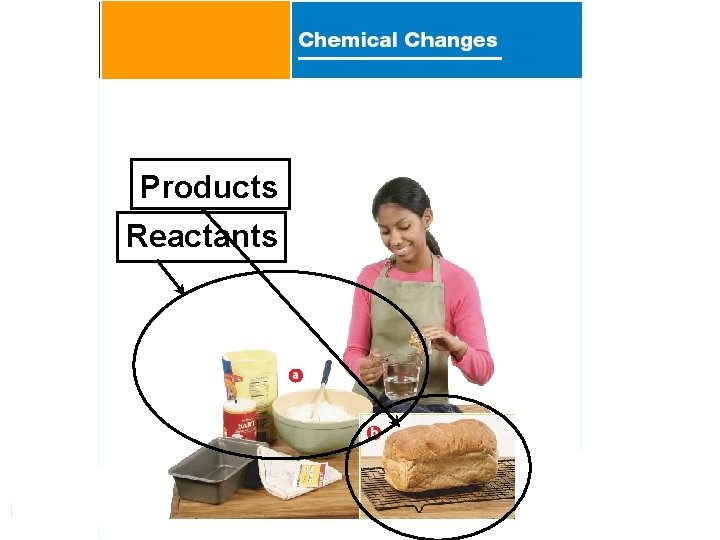- Page 321 Products Reactants 5In a chemical reaction Atoms aren't created or destroyed (according to the Law of Conservation of Mass) A reaction can be described several ways: § § In a sentence every item is a word: Copper reacts with chlorine to form copper (II) chloride. In a word equation some symbols used copper + chlorine copper (II) chloride 6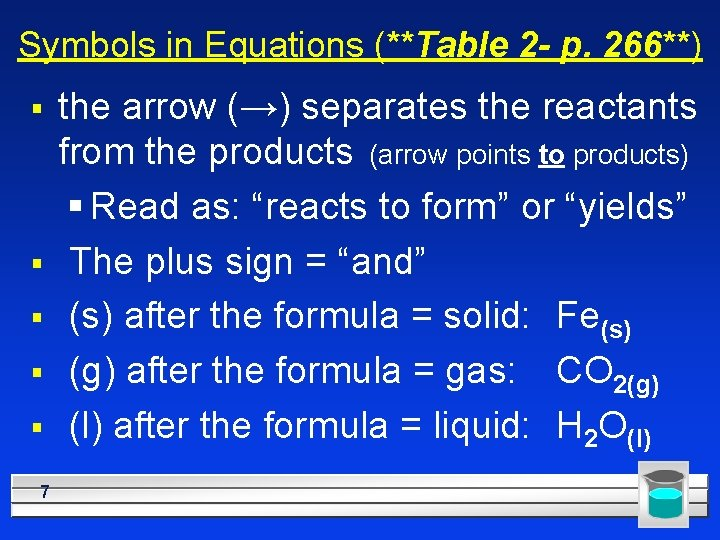Symbols in Equations (**Table 2 - p. 266**) § § § 7 the arrow (→) separates the reactants from the products (arrow points to products) § Read as: “reacts to form” or “yields” The plus sign = “and” (s) after the formula = solid: Fe(s) (g) after the formula = gas: CO 2(g) (l) after the formula = liquid: H 2 O(l)Symbols used in Equations § (aq) after the formula = dissolved in water, an aqueous solution: Na. Cl(aq) is a salt water solution § ↑ used after a product indicates a gas has been produced: H 2↑ § ↓ used after a product indicates a solid has been produced: Pb. I 2↓ 8Symbols used in equations ■ double arrow indicates a reversible reaction (more later) ■ shows that heat is supplied to the reaction ■ is used to indicate a catalyst is supplied (in this case, platinum is the catalyst) 9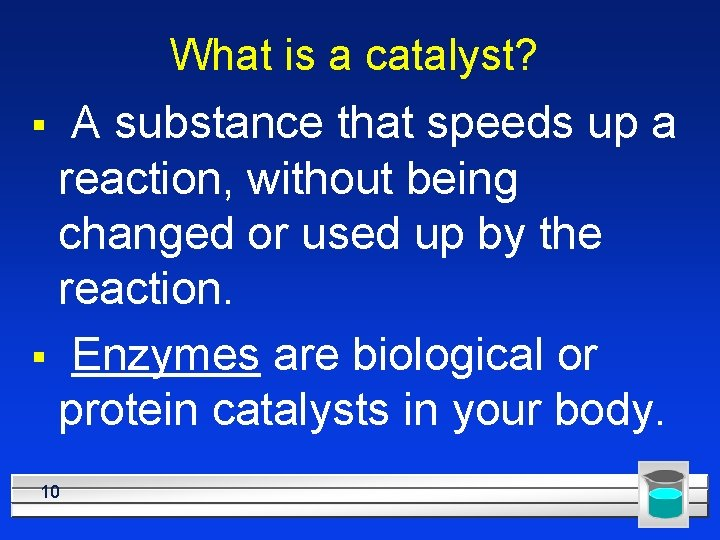What is a catalyst? A substance that speeds up a reaction, without being changed or used up by the reaction. § Enzymes are biological or protein catalysts in your body. § 10The Formula Equation § Uses formulas and symbols to describe a reaction § but doesn’t indicate how many; this means they might NOT be balanced § Chemical equations describe the chemical reaction. 11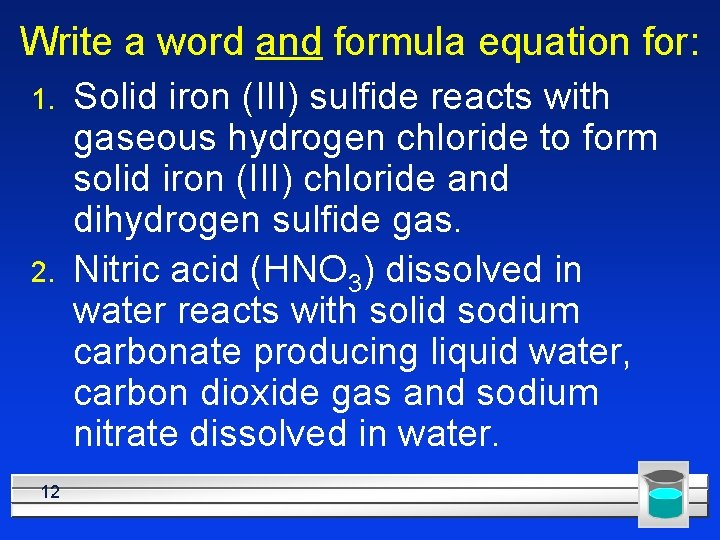Write a word and formula equation for: 1. 2. 12 Solid iron (III) sulfide reacts with gaseous hydrogen chloride to form solid iron (III) chloride and dihydrogen sulfide gas. Nitric acid (HNO 3) dissolved in water reacts with solid sodium carbonate producing liquid water, carbon dioxide gas and sodium nitrate dissolved in water.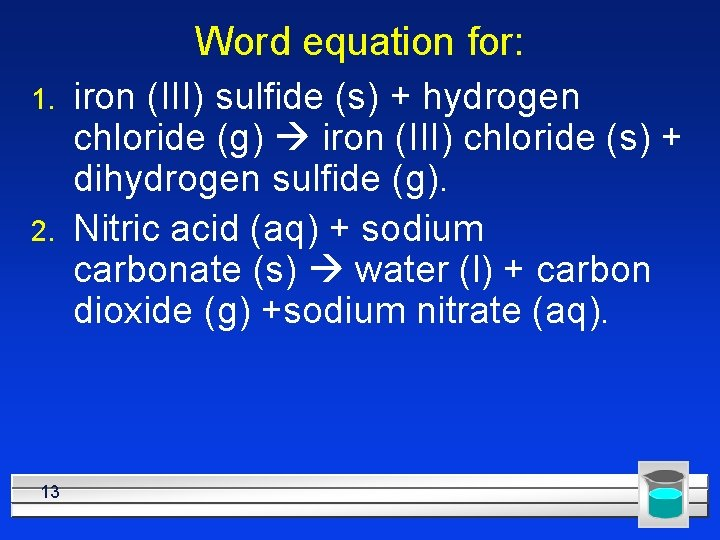Word equation for: 1. 2. 13 iron (III) sulfide (s) + hydrogen chloride (g) iron (III) chloride (s) + dihydrogen sulfide (g). Nitric acid (aq) + sodium carbonate (s) water (l) + carbon dioxide (g) +sodium nitrate (aq).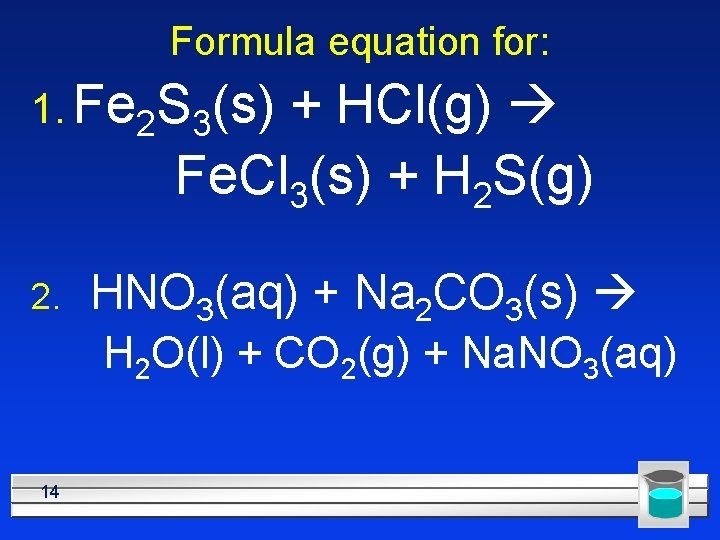Formula equation for: 1. Fe 2 S 3(s) + HCl(g) Fe. Cl 3(s) + H 2 S(g) 2. HNO 3(aq) + Na 2 CO 3(s) H 2 O(l) + CO 2(g) + Na. NO 3(aq) 14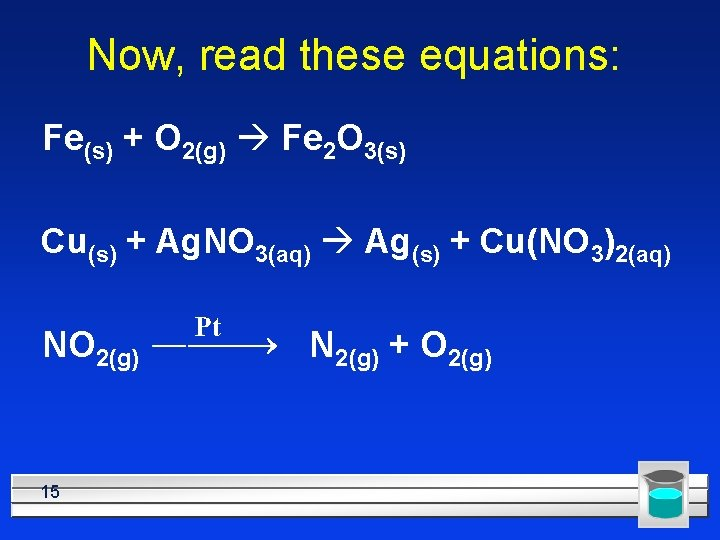Now, read these equations: Fe(s) + O 2(g) Fe 2 O 3(s) Cu(s) + Ag. NO 3(aq) Ag(s) + Cu(NO 3)2(aq) NO 2(g) 15 N 2(g) + O 2(g)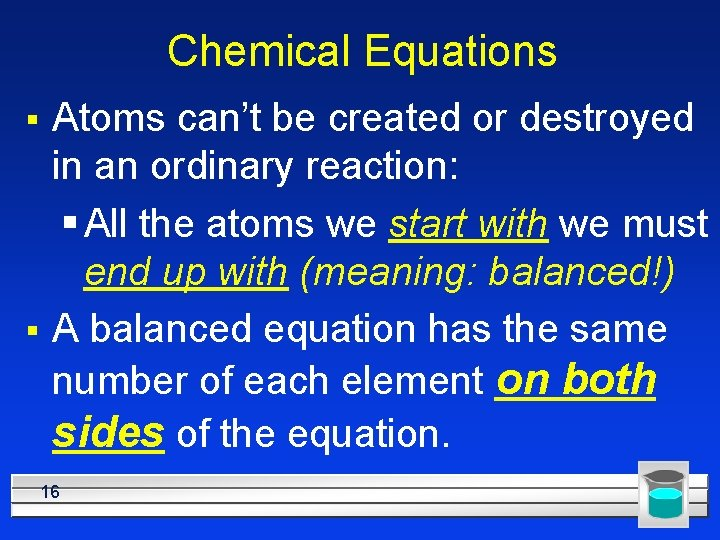Chemical Equations § § Atoms can’t be created or destroyed in an ordinary reaction: § All the atoms we start with we must end up with (meaning: balanced!) A balanced equation has the same number of each element on both sides of the equation. 16Rules for balancing: 1) 2) 3) 4) 4) 17 Assemble the correct formulas for all the reactants and products, using “+” and “→” Count the number of atoms of each type appearing on both sides Balance the elements one at a time by adding coefficients (the numbers in front) where you need more If the reaction contains only C, H, and O, balance in that order. Double-Check to make sure it is balanced.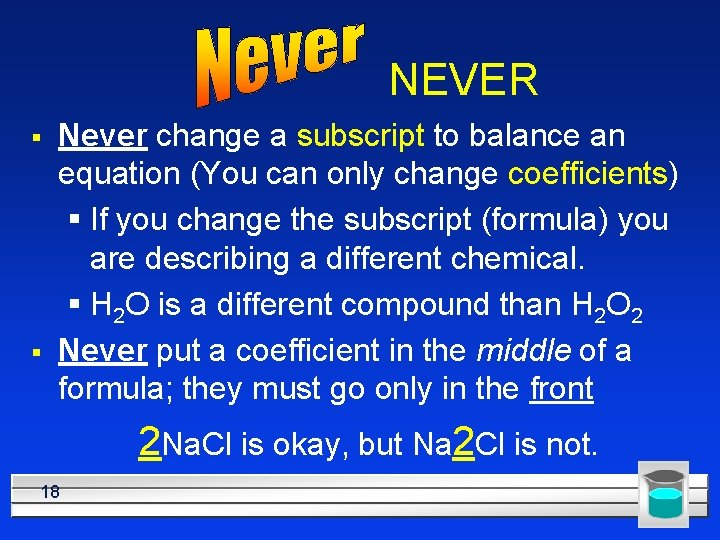NEVER § § Never change a subscript to balance an equation (You can only change coefficients) § If you change the subscript (formula) you are describing a different chemical. § H 2 O is a different compound than H 2 O 2 Never put a coefficient in the middle of a formula; they must go only in the front 2 Na. Cl is okay, but Na 2 Cl is not. 18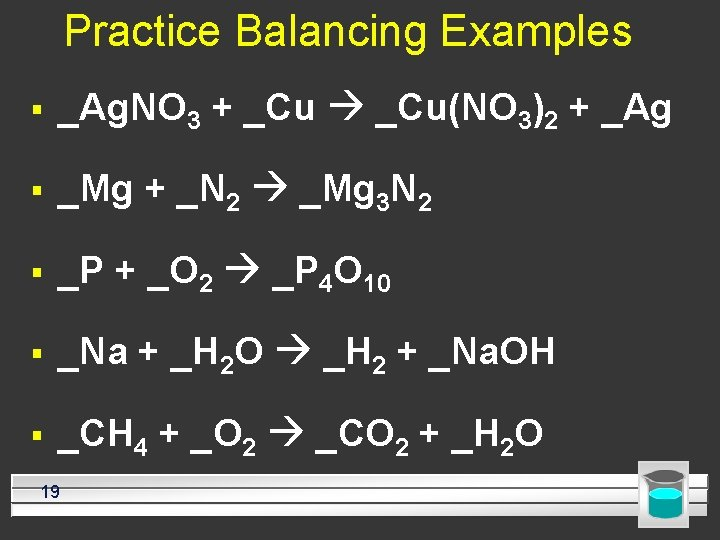Practice Balancing Examples § _Ag. NO 3 + _Cu(NO 3)2 + _Ag § _Mg + _N 2 _Mg 3 N 2 § _P + _O 2 _P 4 O 10 § _Na + _H 2 O _H 2 + _Na. OH § _CH 4 + _O 2 _CO 2 + _H 2 O 19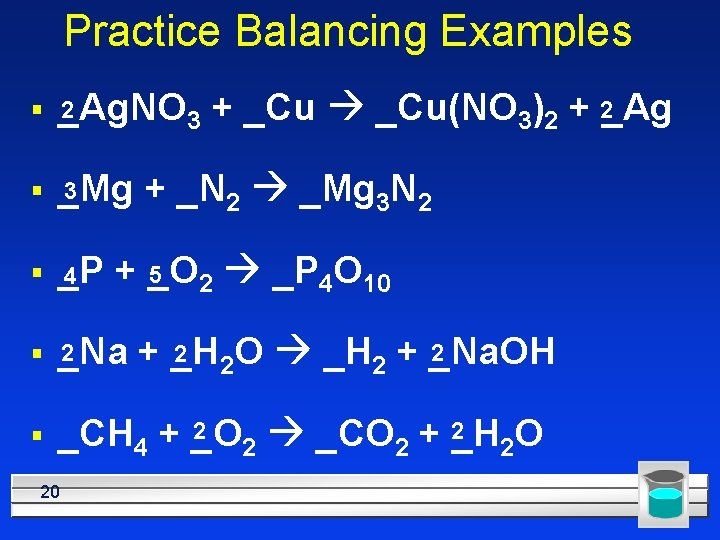Practice Balancing Examples 2 § _Ag. NO 3 3 § _Mg § _P 4 + _N 2 _Mg 3 N 2 5 + _O 2 _P 4 O 10 2 § _Na § + _Cu(NO 3)2 + 2_Ag 2 2 + _H 2 O _H 2 + _Na. OH 2 2 _CH 4 + _O _CO + _H 2 O 2 2 20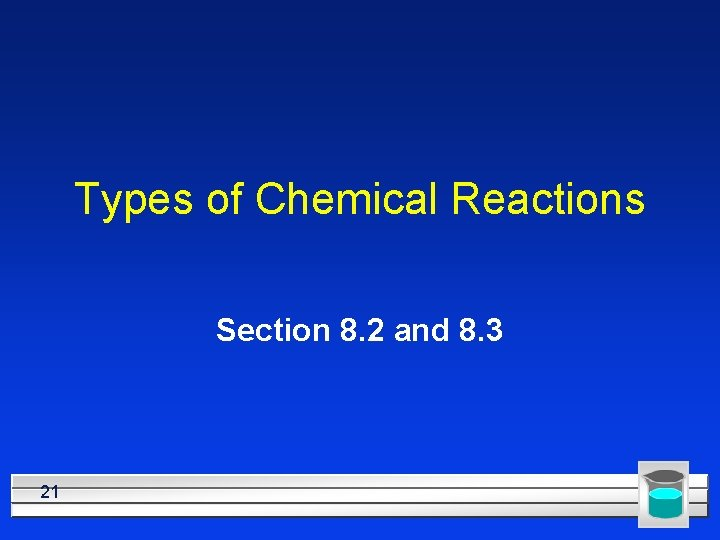Types of Chemical Reactions Section 8. 2 and 8. 3 21Types of Reactions § § § 22 There are probably millions of reactions. We can’t remember them all, but luckily they will fall into several categories. We will learn: a) the 5 major types. We will be able to: b) predict the products. For some, we will be able to: c) predict whether or not they will happen at all#1 – Combination (Synthesis) Reactions § § § Combine = put together Two simpler substances combine to make one more complex compound 2 Ca(s) + O 2(g) 2 Ca. O(s) SO 3(g) + H 2 O(l) H 2 SO 4(aq) We can predict the products, especially if the reactants are two elements. 2 Mg(s) + O 2(g) 2 Mg. O(s)(symbols, charges, cross) 23Complete and Balance: l l l 24 Ca + Cl 2 Fe + O 2 Al + O 2 Remember: the first step is to write the correct formulas Then balance by changing just the coefficients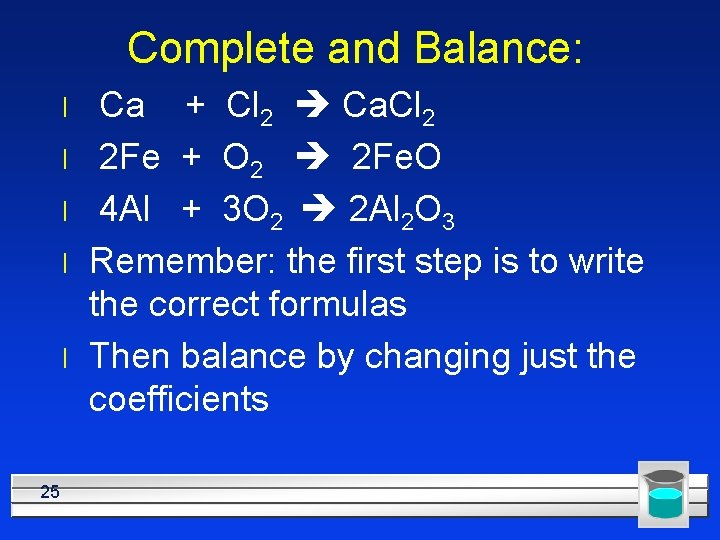Complete and Balance: l l l 25 Ca + Cl 2 Ca. Cl 2 2 Fe + O 2 2 Fe. O 4 Al + 3 O 2 2 Al 2 O 3 Remember: the first step is to write the correct formulas Then balance by changing just the coefficients#1 Combination Reactions l Some nonmetal oxides react with water to produce an acid: SO 3(g) + H 2 O(l) H 2 SO 4(aq) l This is what happens to make acid rain l Some metallic oxides react with water to produce a base: Ca. O(s) + H 2 O(l) Ca(OH)2(aq) l l 26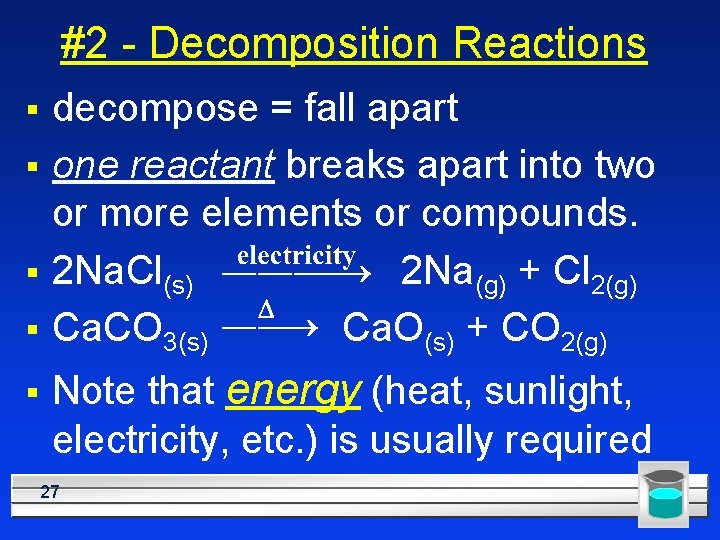#2 - Decomposition Reactions § § § decompose = fall apart one reactant breaks apart into two or more elements or compounds. 2 Na. Cl(s) 2 Na(g) + Cl 2(g) Ca. CO 3(s) Ca. O(s) + CO 2(g) Note that energy (heat, sunlight, electricity, etc. ) is usually required 27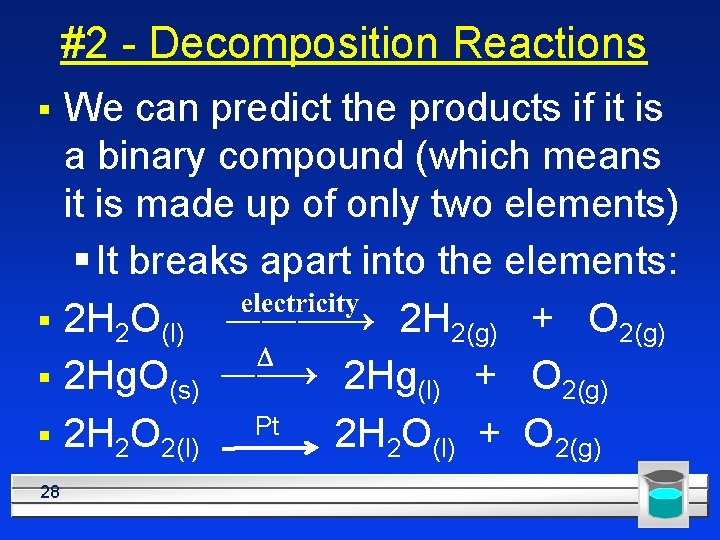#2 - Decomposition Reactions § § 28 We can predict the products if it is a binary compound (which means it is made up of only two elements) § It breaks apart into the elements: 2 H 2 O(l) 2 H 2(g) + O 2(g) 2 Hg. O(s) 2 Hg(l) + O 2(g) 2 H 2 O 2(l) Pt 2 H 2 O(l) + O 2(g)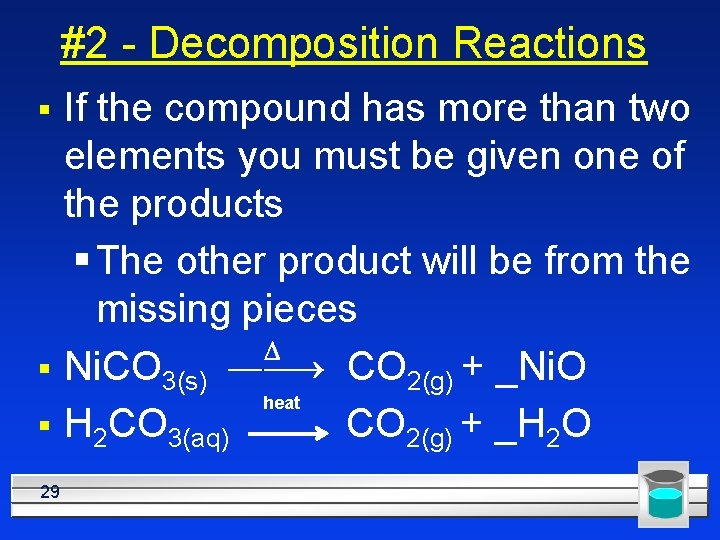#2 - Decomposition Reactions § § § 29 If the compound has more than two elements you must be given one of the products § The other product will be from the missing pieces Ni. CO 3(s) CO 2(g) + _Ni. O heat H 2 CO 3(aq) CO 2(g) + _H 2 O#3 - Single Displacement Reactions § § § One element displaces another Reactants must be an element and a compound. Products will be a different element and a different compound. K + Na. Cl Na + KCl (Cations switched) F 2 + Li. Cl Li. F + Cl 2 (Anions switched) 30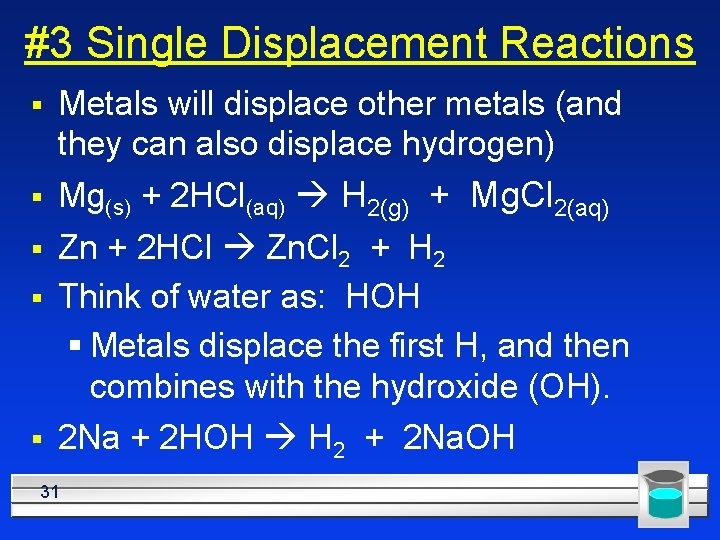#3 Single Displacement Reactions § Metals will displace other metals (and they can also displace hydrogen) § Mg(s) + 2 HCl(aq) H 2(g) + Mg. Cl 2(aq) § Zn + 2 HCl Zn. Cl 2 + H 2 Think of water as: HOH § Metals displace the first H, and then combines with the hydroxide (OH). § § 2 Na + 2 HOH H 2 + 2 Na. OH 31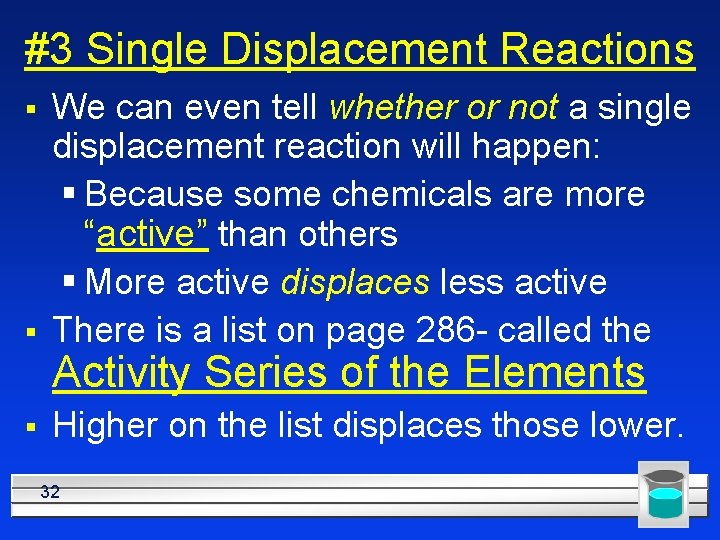#3 Single Displacement Reactions § § We can even tell whether or not a single displacement reaction will happen: § Because some chemicals are more “active” than others § More active displaces less active There is a list on page 286 - called the Activity Series of the Elements § Higher on the list displaces those lower. 32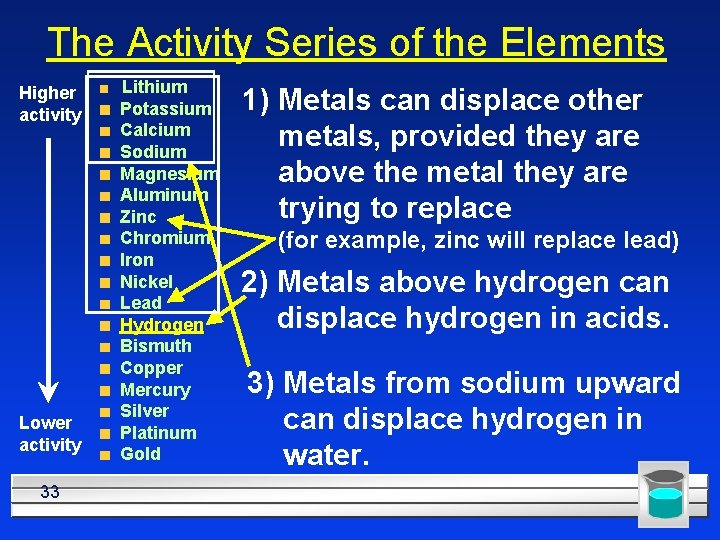The Activity Series of the Elements Higher activity Lower activity 33 Lithium Potassium Calcium Sodium Magnesium Aluminum Zinc Chromium Iron Nickel Lead Hydrogen Bismuth Copper Mercury Silver Platinum Gold 1) Metals can displace other metals, provided they are above the metal they are trying to replace (for example, zinc will replace lead) 2) Metals above hydrogen can displace hydrogen in acids. 3) Metals from sodium upward can displace hydrogen in water.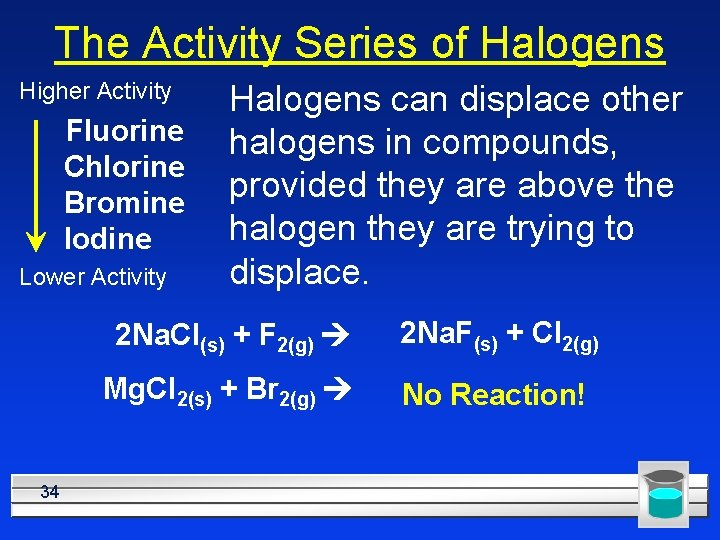The Activity Series of Halogens Higher Activity Fluorine Chlorine Bromine Iodine Lower Activity 34 Halogens can displace other halogens in compounds, provided they are above the halogen they are trying to displace. 2 Na. Cl(s) + F 2(g) 2 Na. F(s) + Cl 2(g) Mg. Cl 2(s) + Br 2(g) No Reaction!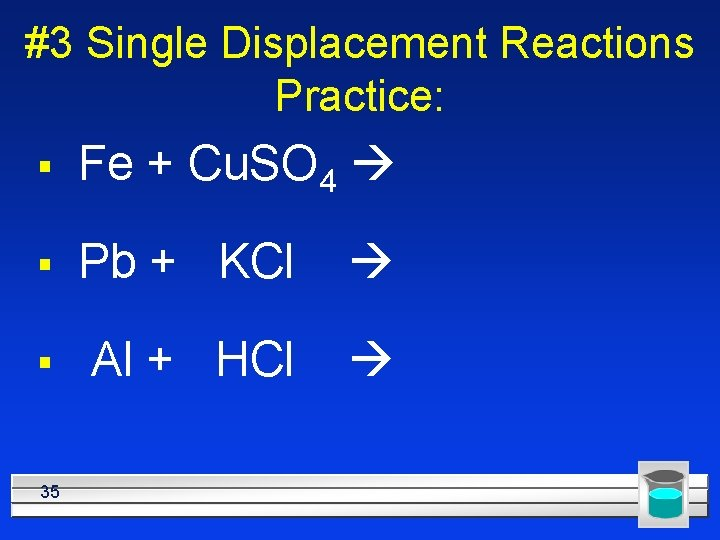#3 Single Displacement Reactions Practice: § Fe + Cu. SO 4 § Pb + KCl § Al + HCl 35#3 Single Displacement Reactions Practice: § Fe + Cu. SO 4 Fe. SO 4 + Cu § Pb + KCl no reaction § 2 Al + 6 HCl 2 Al. Cl 3 + 3 H 2 36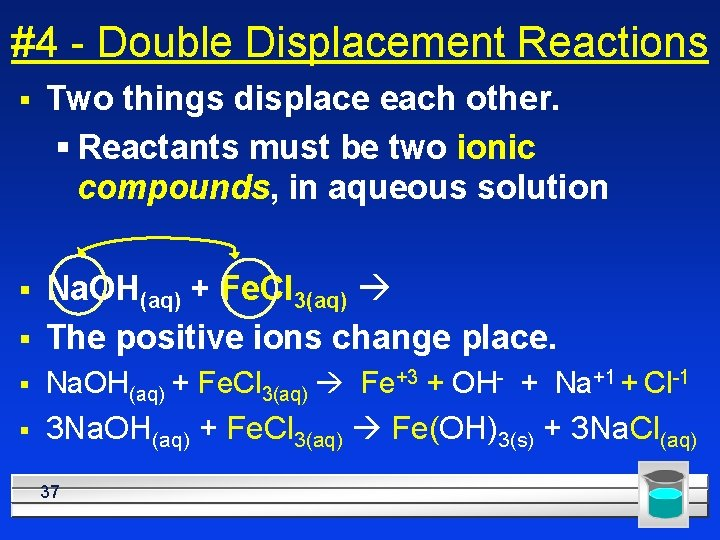#4 - Double Displacement Reactions § Two things displace each other. § Reactants must be two ionic compounds, in aqueous solution § § Na. OH(aq) + Fe. Cl 3(aq) The positive ions change place. § Na. OH(aq) + Fe. Cl 3(aq) Fe+3 + OH- + Na+1 + Cl-1 § 3 Na. OH(aq) + Fe. Cl 3(aq) Fe(OH)3(s) + 3 Na. Cl(aq) 37#4 - Double Displacement Reactions § Have certain “driving forces”, or reasons – Will only happen if one of the products: a) doesn‘t dissolve in water and forms a solid (a “precipitate”), or b) is a gas that bubbles out, or c) is a molecular compound (which will usually be water). 38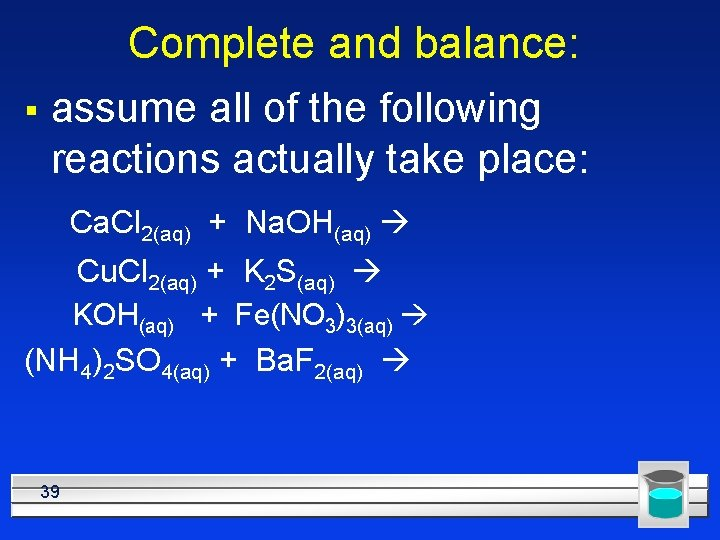Complete and balance: § assume all of the following reactions actually take place: Ca. Cl 2(aq) + Na. OH(aq) Cu. Cl 2(aq) + K 2 S(aq) KOH(aq) + Fe(NO 3)3(aq) (NH 4)2 SO 4(aq) + Ba. F 2(aq) 39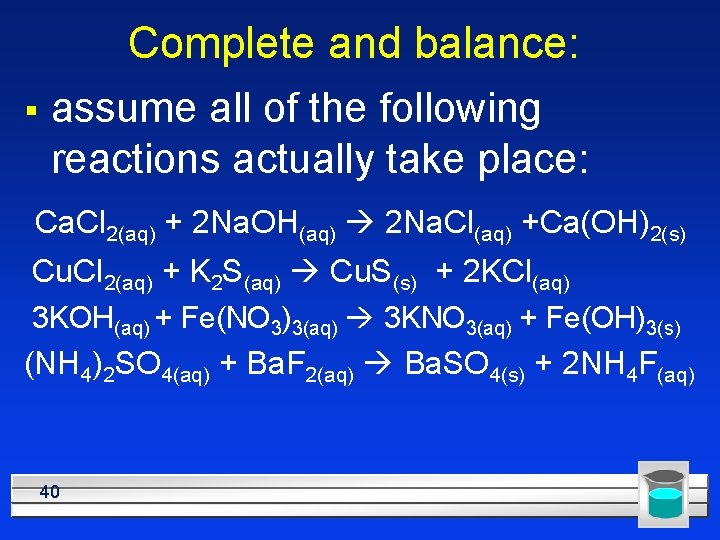Complete and balance: § assume all of the following reactions actually take place: Ca. Cl 2(aq) + 2 Na. OH(aq) 2 Na. Cl(aq) +Ca(OH)2(s) Cu. Cl 2(aq) + K 2 S(aq) Cu. S(s) + 2 KCl(aq) 3 KOH(aq) + Fe(NO 3)3(aq) 3 KNO 3(aq) + Fe(OH)3(s) (NH 4)2 SO 4(aq) + Ba. F 2(aq) Ba. SO 4(s) + 2 NH 4 F(aq) 40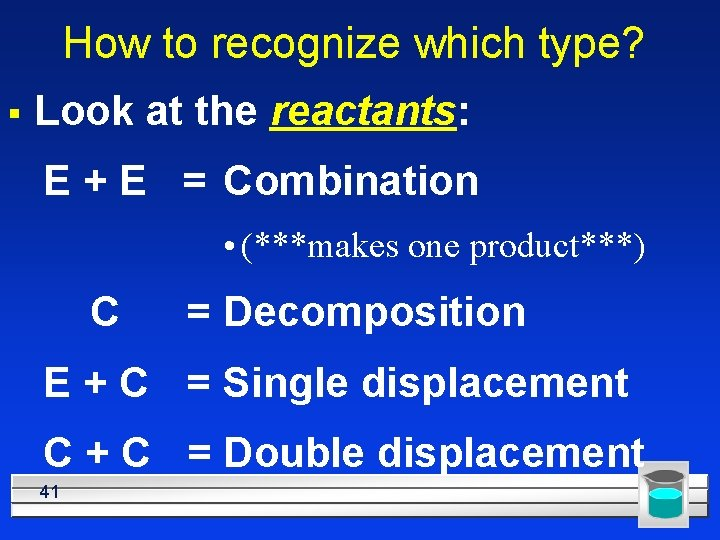How to recognize which type? § Look at the reactants: E + E = Combination • (***makes one product***) C = Decomposition E + C = Single displacement C + C = Double displacement 41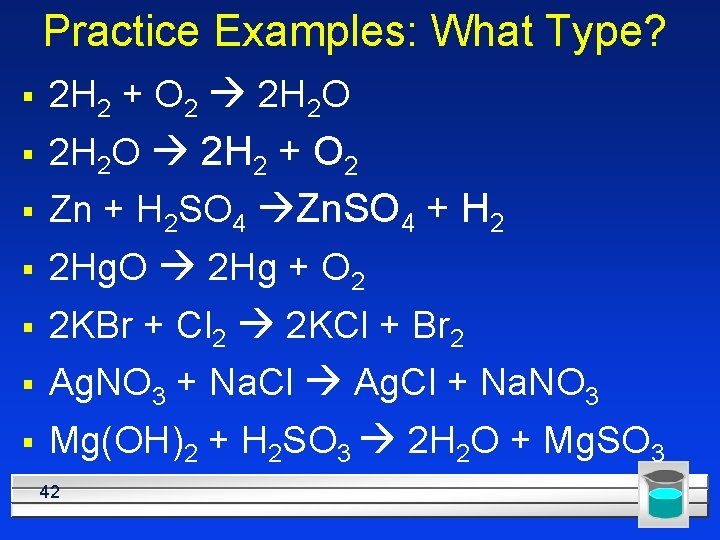Practice Examples: What Type? § 2 H 2 + O 2 2 H 2 O § 2 H 2 O 2 H 2 + O 2 § Zn + H 2 SO 4 Zn. SO 4 + H 2 § 2 Hg. O 2 Hg + O 2 § 2 KBr + Cl 2 2 KCl + Br 2 § Ag. NO 3 + Na. Cl Ag. Cl + Na. NO 3 § Mg(OH)2 + H 2 SO 3 2 H 2 O + Mg. SO 3 42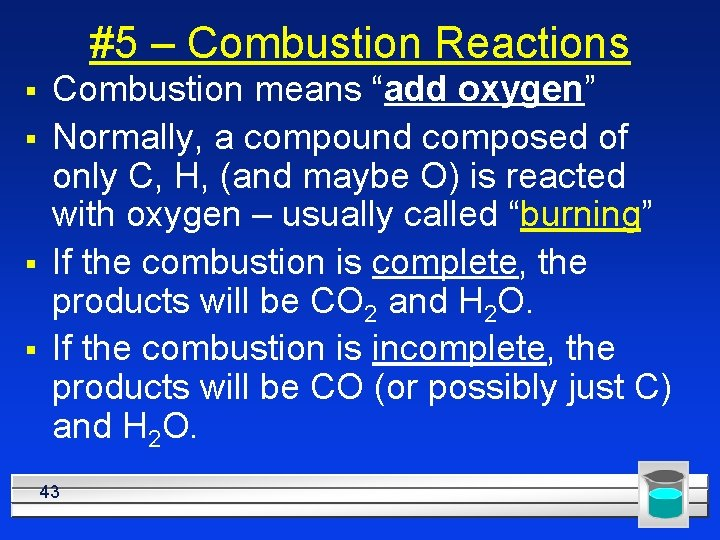#5 – Combustion Reactions § § Combustion means “add oxygen” Normally, a compound composed of only C, H, (and maybe O) is reacted with oxygen – usually called “burning” If the combustion is complete, the products will be CO 2 and H 2 O. If the combustion is incomplete, the products will be CO (or possibly just C) and H 2 O. 43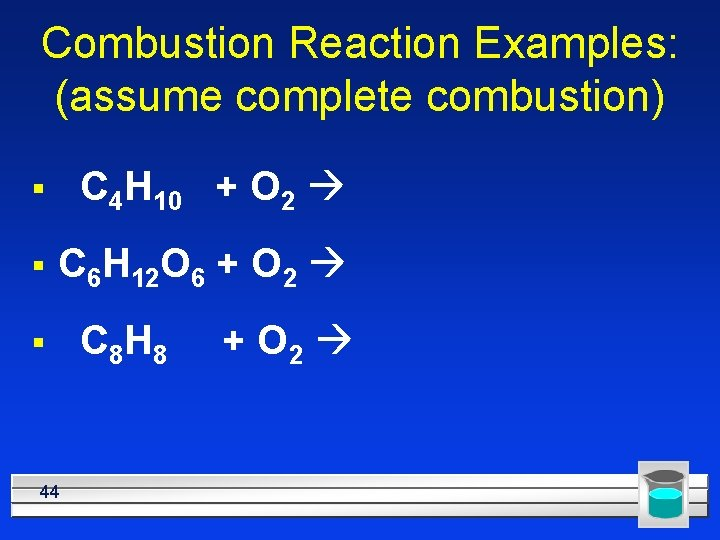Combustion Reaction Examples: (assume complete combustion) § C 4 H 10 + O 2 § C 6 H 12 O 6 + O 2 § 44 C 8 H 8 + O 2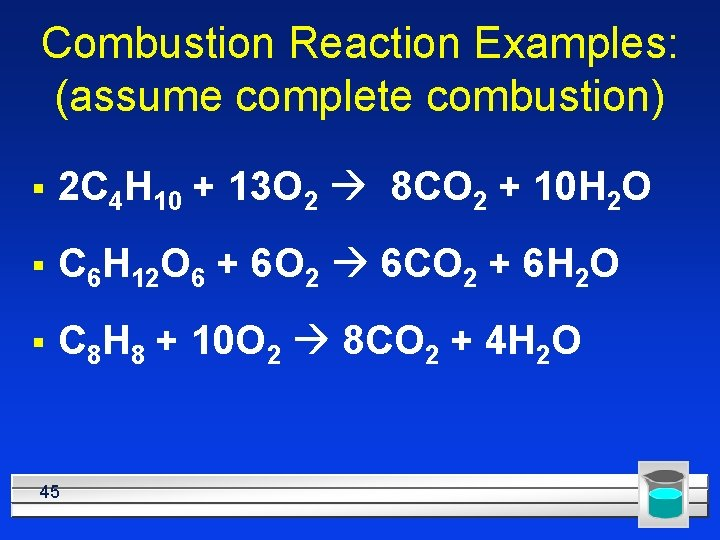Combustion Reaction Examples: (assume complete combustion) § 2 C 4 H 10 + 13 O 2 8 CO 2 + 10 H 2 O § C 6 H 12 O 6 + 6 O 2 6 CO 2 + 6 H 2 O § C 8 H 8 + 10 O 2 8 CO 2 + 4 H 2 O 45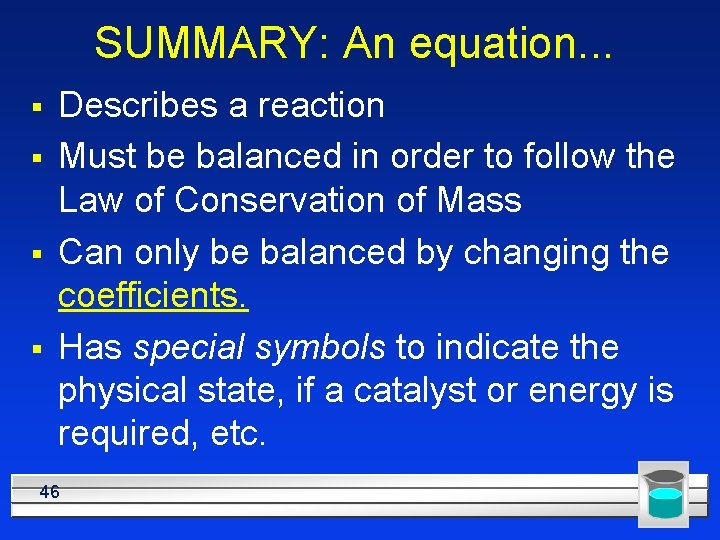SUMMARY: An equation. . . § § Describes a reaction Must be balanced in order to follow the Law of Conservation of Mass Can only be balanced by changing the coefficients. Has special symbols to indicate the physical state, if a catalyst or energy is required, etc. 46Reactions § § Come in 5 major types. We can tell what type they are by looking at the reactants/products. Single displacement happens based on the Activity Series Double displacement happens if one product is: 1) a precipitate (an insoluble solid), 2) water (a molecular compound), or 3) a gas. 47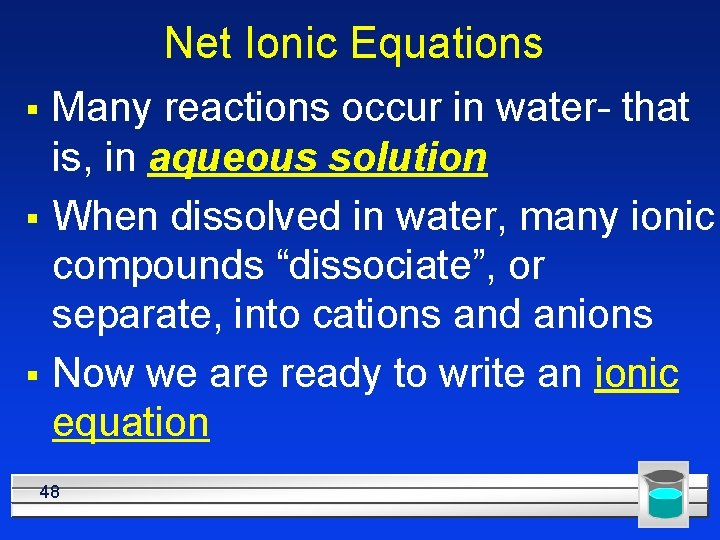Net Ionic Equations § § § Many reactions occur in water- that is, in aqueous solution When dissolved in water, many ionic compounds “dissociate”, or separate, into cations and anions Now we are ready to write an ionic equation 48Net Ionic Equations Example (needs to be a double displacement reaction) Ag. NO 3(aq) + Na. Cl(aq) Ag. Cl(s) + Na. NO 3(aq) 1. this is the full balanced equation 2. next, write it as an ionic equation by splitting the compounds into their ions: § Ag+ + NO 3 - + Na+ + Cl- Ag. Cl + Na+ + NO 3 Note that the Ag. Cl did not ionize, because it is a precipitate 49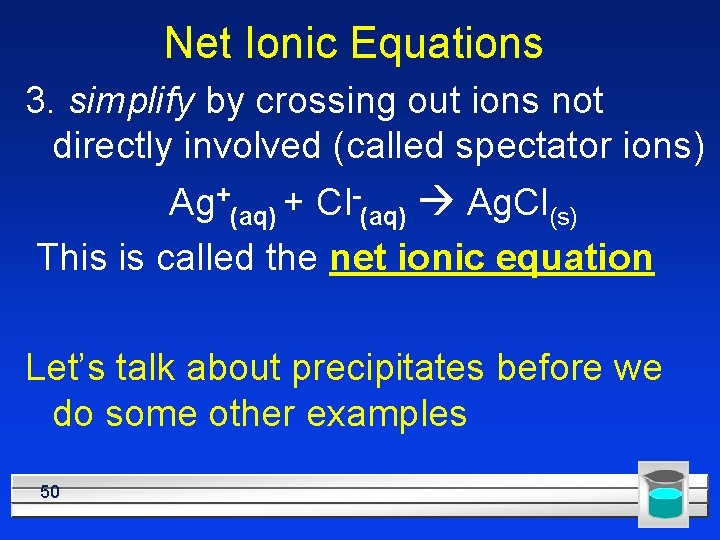Net Ionic Equations 3. simplify by crossing out ions not directly involved (called spectator ions) Ag+(aq) + Cl-(aq) Ag. Cl(s) This is called the net ionic equation Let’s talk about precipitates before we do some other examples 50Predicting the Precipitate § § § General solubility rules (also for double displacement reactions) Always soluble: salts of Na+, K+, NH 4+, and NO 3 Usually soluble: salts of Cl- and SO 42 -, but Ag. Cl, Pb. Cl 2, Pb. SO 4 and Ba. SO 4 are insoluble Usually insoluble: salts of OH-, O 2 -, CO 32 -, and PO 43 - (unless with Na+, K+, or NH 4+) Slightly soluble: Ca(OH)2 and Ca. SO 4 51Solubility Rules • Not all ionic compounds are soluble in water. We can use the solubility rules to predict if a compound will be soluble in water. © 2014 Pearson Education, Inc. Chapter 7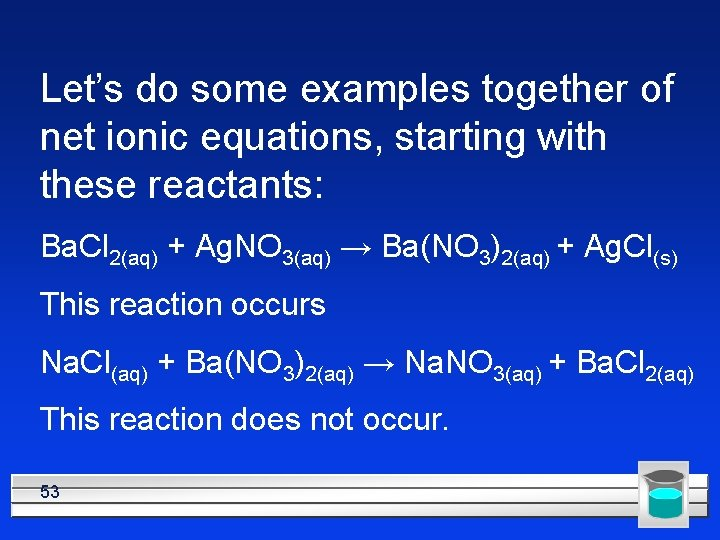Let’s do some examples together of net ionic equations, starting with these reactants: Ba. Cl 2(aq) + Ag. NO 3(aq) → Ba(NO 3)2(aq) + Ag. Cl(s) This reaction occurs Na. Cl(aq) + Ba(NO 3)2(aq) → Na. NO 3(aq) + Ba. Cl 2(aq) This reaction does not occur. 53